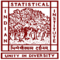Histogram vs. Bar Graph: What is the Difference?

# Histogram vs. Bar Graph: What is the Difference?

clickHere
Updated on Sep 26, 2022 19:05 IST

In this blog on histogram vs. bar graph, find out the definitions, parts and types of each.

Histograms and bar graphs may look identical, but they are different from each other. Visually, a histogram has adjacent columns, while in a bar graph, there are spaces between the bars. This is the first point of visual distinction between the two. Today, the blog will cover the histogram vs. bar graph debate in some more detail.

## What is a Histogram?

Used mostly in statistics, histograms represent the frequency distribution of a continuous dataset. The frequency distribution is created by classes or bins, a range of values comprising data points.

Histograms are used when the range of continuous data is binned/classed into intervals that appear as a series. Statisticians then count the values in each interval. A histogram is also used as a 7 QC tool

### Parts of a Histogram

In the histogram, there are four parts.

• Title – It describes what is included in the histogram
• X-axis – The X-axis represents the intervals under which the values are measured
• Y-axis – It shows the number of times the values (or value) occur within the intervals along the X-axis
• Bars (Height & Width) – The height of the bar determines how many times the value occurs in the interval. The width corresponds to the equal class intervals.

### Types of Histograms

Some of the most popular types of histograms are

#### Normal Distribution

Also known as Gaussian distribution, it appears symmetrical, where the points of one side of the average are equivalent to the point of the other.

The maximum number of values will be close to the mean and the minimum number away from the mean, both left and right.

Must read: Difference between mean and median

For instance, you want to look up the values of heights of males in a specific location. The mean is 6 feet. The shorter heights will start at the histogram’s left and gradually go up to the mean. Then as you go towards the right, the height of males is more than the mean, and they will gradually go down the right. The frequency will determine how many people are 6 feet and how many of those are less than or greater than 6 feet in height.

#### Bimodal Distribution

A histogram shows two peaks when it has a bimodal distribution.

Bimodal distribution is used for analysing two groups. If you take the same example from above, for a bimodal distribution there would be an additional analysis. Apart from the heights of males in a particular area, there would be the height of females in the same area. Here the mean can be 5.8 feet. The frequency of females close to this mean would be there and the number of females who are less than those in the mean will be shown on two opposite sides.

## What is a Bar Graph?

A bar graph is also known as a bar chart. The rectangular bars are separated but at equal distances from each other.

Unlike a histogram that groups continuous data, a bar graph is a graphical representation of categorical data.

### Parts of a Bar Graph

• Title: It tells what the graph is about
• Scale: The numbers that show the units on the graph
• Label: They exist on both along the x-and y-axes. Typically, the label on the x-axis determines the type of data on the line, while on the y-axis, the label is the numeric value for each point.

### Types of Bar Graph

There are four types of bar graphs.

#### Horizontal Bar Graphs

Here, the rectangular bars are drawn across the y-axis. The length of the bars is shown along the x-axis.

#### Vertical Bar Graphs

The rectangular bars are drawn on the x-axis, and the length of the bar is along the y-axis.

#### Grouped Bar Graphs

It is used for visualizing discrete data of more than two categorical data. The representation of data in grouped bar graphs can be shown horizontally or vertically.

#### Stacked Bar Chart

This type of bar chart categorises the whole bar into different parts with different colours to represent them. It can be shown vertically or horizontally.

## Histogram Vs. Bar Charts

Here is a comparison table between a histogram and a bar chart.

## Parting Thoughts

Understanding the histogram vs. bar graph debate makes you know how to visualise data in appropriate ways. Hope the above explanations helped you determine the difference between the two. Also, check out the top 10 data visualization tools.

clickHere

Aquib is a seasoned wordsmith, having penned countless blogs for Indian and international brands. These days, he's all about digital marketing and core management subjects - not to mention his unwavering commitment ... Read Full Bio

## Trending Data Science Courses## Top Picks & New Arrivals